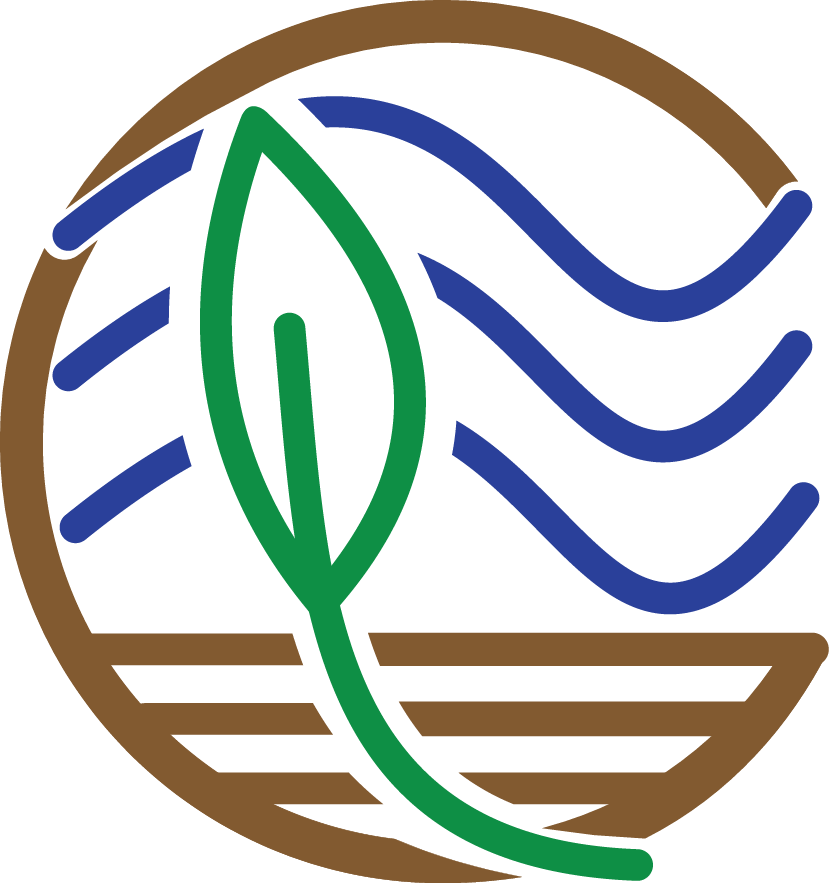# Plot Maps# Plot Maps

Let’s finish by creating more visuals; this time, a series of maps. The core_data tibble includes longitude and latitude of each soil core, so we can use those coordinates to plot where the cores were taken in geographical space:

    # Plot cores on a lat/long coordinate plane
map <- ggplot() +
geom_point(data = core_data, aes(x = core_longitude, y = core_latitude)) + # Add coordinate data
theme_bw() + # Change the plot theme
ggtitle("Distribution of Sampled Cores across CONUS") + # Give the plot a title
xlab("Longitude") + # Change x axis title
ylab("Latitude") # Change y axis title
mapNot bad, but we can do more. Let’s plot a coordinate display of our cores overlayed on top of a map of the conterminous US, and display cores by a color gradient corresponding to the fraction of organic matter. We’ll use many of the operations that we learned in this tutorial all in sequence.

We’re also going to integrate a few packages that help with processing and displaying spatial data, rgdal and ggmap.

    # Load new packages
devtools::install_github("dkahle/ggmap") # As of time of writing, this is a package that receives frequent
# updates. So it's good to install from GitHub
library(ggmap)
library(rgdal)

# Join core_data and depthseries_data
core_depth_data <- left_join(core_data, depthseries_data, by = NULL)

# Select coordinate data from core_data and redefine into new dataset
core_coordinates <- select(core_depth_data, core_longitude, core_latitude, fraction_organic_matter)

CONUS_map <- get_map(location = c(lon = -96.5795, lat = 39.8283), # center map on CONUS
color = "color",
maptype = "terrain-background",
zoom = 4) # set the zoom to include all data

# Add the CONUS_map with axes set as Longitude and Latitude
ggmap(CONUS_map,
extent = "device",
xlab = "Longitude",
ylab = "Latitude") +

# Add core lat/long coordinates as points, colored by fraction organic matter
geom_point(data = core_coordinates, aes(x = core_longitude, y = core_latitude, color =
fraction_organic_matter), alpha = 1, size = 1) +
colors = c("#EFEC35", "#E82323"), na.value = "#1a1a1a") + # Provide ggplot2 with color gradient
ggtitle("Distribution of Sampled Cores across CONUS") + # Give the plot a title
xlab("Longitude") + # Change x axis title
ylab("Latitude") # Change y axis title# Filter to specific regions for map insets

# Add inset of Coastal Louisiana
LA_map <- get_map(location = c(lon = -91.0795, lat = 30.2283),
color = "bw",
maptype = "satellite",
zoom = 7)

# Add the LA_map with axes set as Longitude and Latitude
ggmap(LA_map,
extent = "device",
xlab = "Longitude",
ylab = "Latitude") +

# Add core lat/long coordinates as points, colored by fraction organic matter
geom_point(data = core_coordinates, aes(x = core_longitude, y = core_latitude, color =
fraction_organic_matter), alpha = 1, size = 2) +
colors = c("#EFEC35", "#E82323"), na.value = "#1a1a1a") # Provide ggplot2 with color gradient# Add inset of Mid-Atlantic Seaboard
mid_Atlantic_map <- get_map(location = c(lon = -75.095, lat = 39.2283),
color = "bw",
maptype = "satellite",
zoom = 7)

# Add the mid_Atlantic_map with axes set as Longitude and Latitude
ggmap(mid_Atlantic_map,
extent = "device",
xlab = "Longitude",
ylab = "Latitude") +

# Add core lat/long coordinates as points, colored by fraction organic matter
geom_point(data = core_coordinates, aes(x = core_longitude, y = core_latitude, color =
fraction_organic_matter), alpha = 1, size = 3) +
colors = c("#EFEC35", "#E82323"), na.value = "#1a1a1a") # Provide ggplot2 with color gradientWhen combined, these maps can tell a lot about soil carbon across the contiguous United States.Website Section
Coastal Carbon